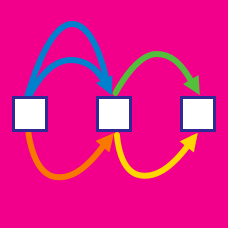Probability

# Rule of Sum and Rule of Product Warmup

A palindrome is a number that reads the same forwards and backwards (like 747). Which of the following numbers is a palindrome?

How many 3-digit palindromes are there? (Note: The first digit of a 3-digit number must be nonzero.)

If $A$ is the number of 3-digit palindromes and $B$ is the number of 4-digit palindromes, which is greater?

How many 3-digit palindromes are there where the middle digit is larger than the outer digits (like 121)?

How many 3-digit palindromes are there where the middle digit is the same as the leading digit?

×